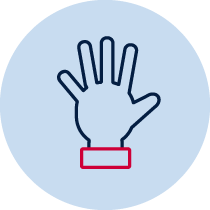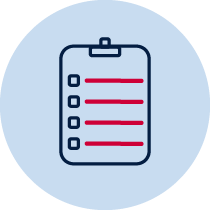# Length – Who wins?

Gauge students’ understanding of initial length concepts.Practical Resource required Teacher observation Individual

## Measurement – length

A student:

• measures, records, compares and estimates lengths and distances using uniform informal units, metres and centimetres MA1-9MG
• supports conclusions by explaining or demonstrating how answers were obtained MA1-3WM
• describes mathematical situations and methods using everyday and some mathematical language, actions, materials, diagrams and symbols MA1-1WM

## Content

• Measure and compare the lengths of pairs of objects using informal uniform units (ACMMG019)
• Compare and order several shapes and objects based on length, using appropriate uniform informal units (ACMMG037)

• Represent and solve simple addition and subtraction problems using a range of strategies, including counting on, partitioning and rearranging units MA1-5NA

## National Numeracy Learning Progression mapping to the NSW mathematics syllabus

When working towards the outcome MA1‑9MG the sub-elements (and levels) of understanding units of measurement (UuM3-UuM5) describe observable behaviours that can aid teachers in making evidence-based decisions about student development and future learning.

## Materials

• paper (one A4 size)
• pencil
• units (paperclips, matchsticks)

## Teacher instructions

The purpose of this task is to gauge students’ understanding of initial length concepts such as:

• estimating and comparing two different lengths
• using uniform informal units to measure the length by placing the units end-to-end without gaps or overlaps

Students will answer the question independently. They will have access to a piece of A4 paper and items to measure, such as matchsticks or paper clips to measure the length of the sides of paper.

The teacher may read the question to the students and record answers if required.

Prompt students to use items to check their answer, eg. paper clips.

Students may draw the different routes the beetles have taken.

The teacher may record observations and how the student explains their thinking.

## Student instructions

One beetle walked around two sides of an A4 piece of paper, and her friend walked diagonally across the paper.

• Which beetle walked the furthest?
• Can you check your answer using the paper clips or matchsticks?
• Can you find a different way for the beetle to go?

## Possible areas for further exploration?

Understanding of the terms, ‘diagonally’ and ‘furthest’ may need clarification. Also may need to discuss the term ‘distance’ for length when talking about travelling from one point or place to another.

Check for alignment of units – end to end without gaps or overlaps. Are the units of equal size?

Does the student know that lengths (not marks or spaces) are counted?

Does the student know that the length is conserved if rearranged?

## Where to next?

Students can experiment with selecting another appropriate informal unit to measure the length and compare which unit is more efficient.

Students may move from informal to formal units of measurement. Discuss other ways we could measure the length and introduce the language of centimetre and metre.

Adapted from Teaching Measurement: Early Stage 1 – Stage 1